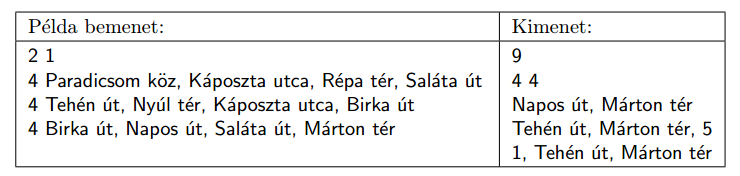Mathematical and Physical Journal
for High Schools
Issued by the MATFUND Foundation
 Already signed up? New to KöMaL?

#Problem I. 355. (October 2014)

I. 355. A certain city has $\displaystyle M$ metro lines and $\displaystyle V$ tram lines. Each of these lines is an ordered set of stops, and one can travel in both directions along each line. It is possible to change from one line to the other one if they share a stop with the same name. You should answer the following questions.

$\displaystyle a)$ Give the number of stops in the city.

$\displaystyle b)$ Give the number of stops of the longest metro and longest tram line.

$\displaystyle c)$ List those stops which cannot be reached by using only metro lines.

$\displaystyle d)$ List two stops having the largest distance and also give this distance. The distance is defined as the number of stops between them by using the shortest route.

$\displaystyle e)$ Suppose we want to get from an arbitrarily given stop to another one by changing lines as few times as possible, then what is the minimal number of changing lines? You should specify the number of changing lines, then give two stops such that it is impossible to get from one to the other by changing lines fewer times than this.

Your program should read the values of $\displaystyle M$ and $\displaystyle V$ ($\displaystyle 1\le M, V\le 100$) from the first line of he standard input. The following $\displaystyle M$ lines then specify the metro lines: an integer at the beginning of each line describes the number of stops of that line, then the stop names are listed (each separated by a comma and a space). The next $\displaystyle V$ lines contain an analogous description of the tram lines. Your program should write the answers to the above 5 questions in the first 5 lines of the standard output. In the example, Példa bemenet'' and Kimenet'' are a sample input and the corresponding output.The source code (i355.pas, i355.cpp, ...) of your program without the .exe or any other auxiliary files generated by the compiler but with a brief documentation (i355.txt, i355.pdf, ...) of your solution should be submitted in a compressed file i355.zip, also specifying the name of the developer environment to use for compiling your source.

(10 pont)

Deadline expired on November 10, 2014.

### Statistics:

 15 students sent a solution. 10 points: Kovács 246 Benedek, Mócsy Miklós, Nagy Ábel, Olexó Gergely. 9 points: Dombai Tamás. 7 points: 1 student. 6 points: 5 students. 5 points: 1 student. 4 points: 1 student. 3 points: 1 student. 1 point: 1 student.

Problems in Information Technology of KöMaL, October 2014